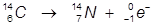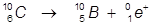# Beta Decay

Radioactive decay involves the spontaneous splitting of heavy unstable isotopes. The isotope splits to create two or more stable particles. One of the three main types of radioactive decay is known as beta decay (β-decay).

During beta decay, a proton in the nucleus of the unstable atom is changed into a neutron or vice-versa. If a proton is converted into a neutron it is known as β+ decay, if a neutron is converted into a proton it is referred to as β- decay.

Due to the change in the nucleus a beta particle is emitted. A beta particle is typically a high speed electron in β- decay or positron (an electron with a positive charge) in β+ decay.

Examples:

1. The β- decay of carbon-14.In this example, a neutron of carbon is converted into a proton and the emitted beta particle is an electron.

2. The β+ decay of carbon-10.In this example, a proton of carbon is converted into a neutron and the emitted beta particle is a positron.

 Related Links: Chemistry Nuclear Chemistry Alpha Decay Gamma Decay Nuclear Fusion Nuclear Reactions

To link to this Beta Decay page, copy the following code to your site: